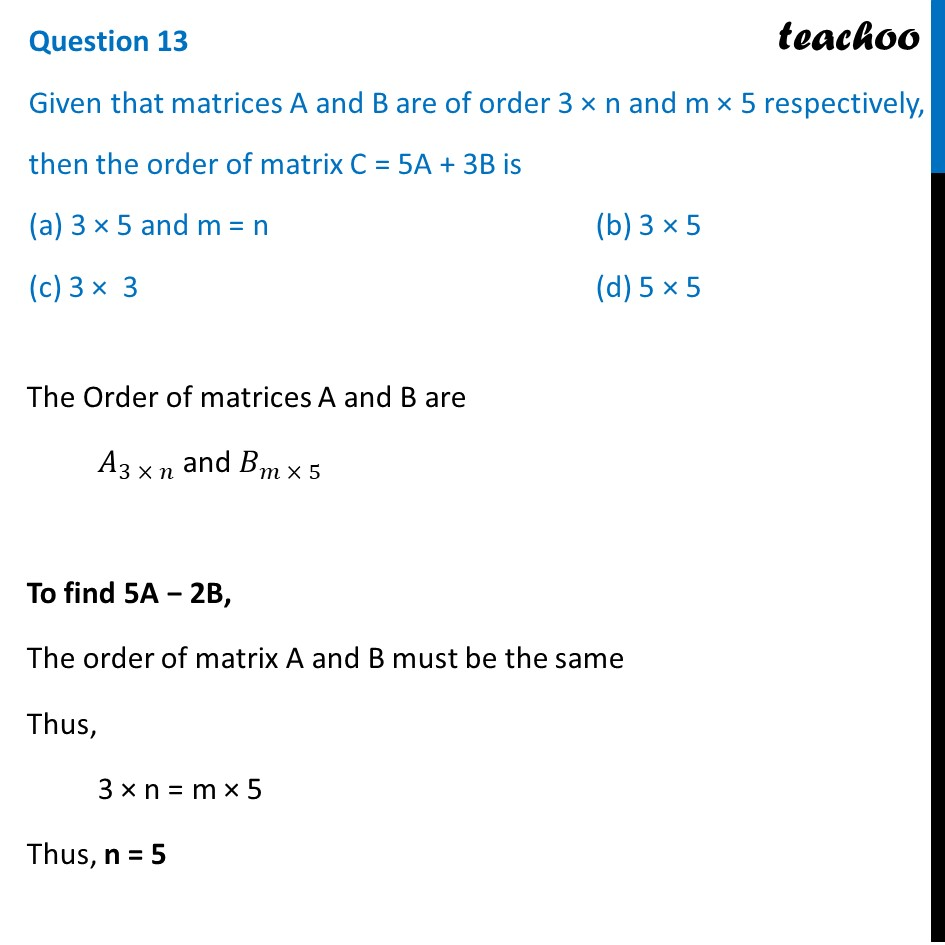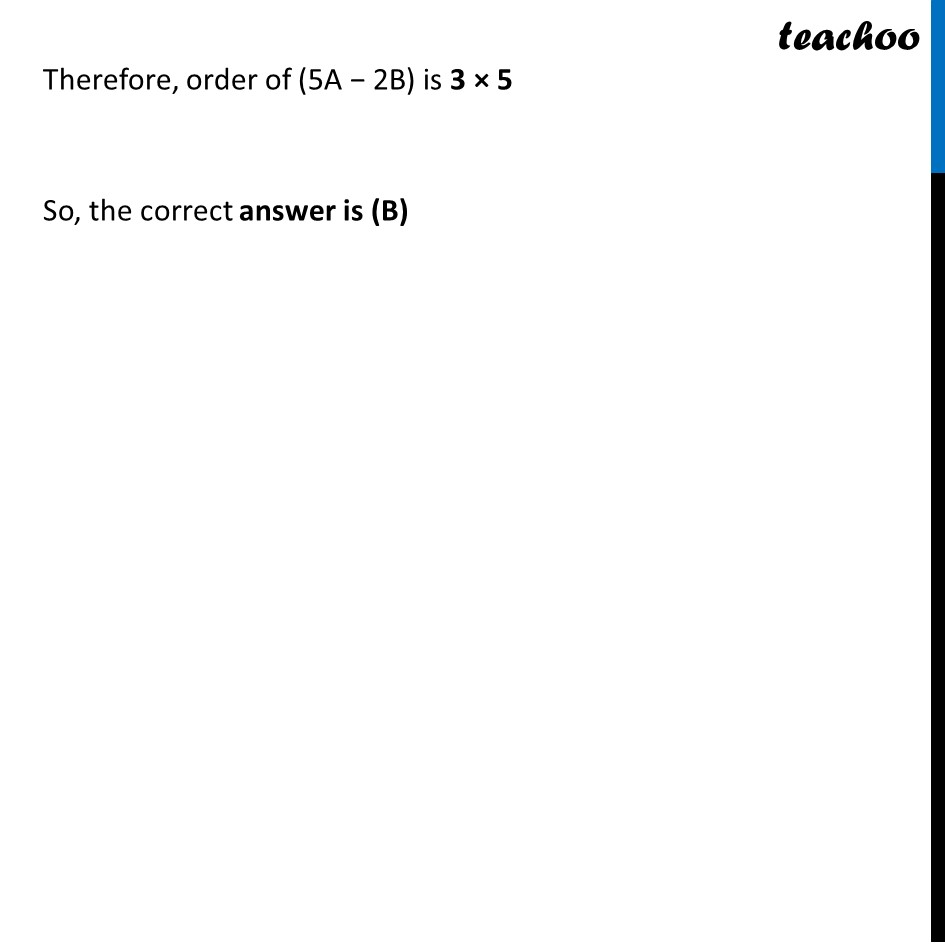CBSE Class 12 Sample Paper for 2022 Boards (MCQ Based - for Term 1)

Class 12
Solutions of Sample Papers and Past Year Papers - for Class 12 Boards

## (a) 3 × 5 and m = n    (b) 3 × 5 (c) 3 ×  3                    (d) 5 × 5

This question is inspired from Question 4 - CBSE Class 12 Sample Paper for 2021 BoardsLearn in your speed, with individual attention - Teachoo Maths 1-on-1 Class

### Transcript

Question 13 Given that matrices A and B are of order 3 × n and m × 5 respectively, then the order of matrix C = 5A + 3B is (a) 3 × 5 and m = n (b) 3 × 5 (c) 3 × 3 (d) 5 × 5 The Order of matrices A and B are 𝐴_(3 × 𝑛) and 𝐵_(𝑚 × 5) To find 5A − 2B, The order of matrix A and B must be the same Thus, 3 × n = m × 5 Thus, n = 5 Therefore, order of (5A − 2B) is 3 × 5 So, the correct answer is (B)